# Standard Atmosphere in R

#### Air Mass Coefficient

The air mass coefficient defines the path length (or column depth) of sunlight through the atmosphere.  The air mass for dry air, wet air and dust are key inputs for estimating solar extinction and the irradiance intensity on the Earth’s surface.  The air mass coefficient is a ratio between the path length for a specific zenith angle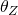and the column depth when the zenith angle equals zero.

(1)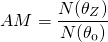The challenge in modeling air mass and solar extinction is an atmosphere that is highly variable, exhibiting unique behavior at different altitude levels.  Atmospheric models seek to overcome some of the errors in the interpolative models of air mass.  Specifically, atmospheric models:

• Define a non-homogenous atmosphere with temperature, pressure and air density profiling by elevation;
• Estimate the column depth of dry air using real-time or forecast data;
• Estimate the column depth of water vapor and droplets; and
• Estimate the column depth of dust and other aerosol concentrations.

The practical goal is to use the latest measurement data and the best atmospheric models to  understand the solar resource and the performance of solar PV technology.

#### Variable Density Atmosphere In the real atmosphere, air density varies with temperature and pressure as a function of height.  The column depth or path length through the dry atmosphere is:

(2)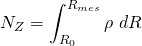where R is the distance from the center of the Earth for the observer at altitude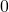and for the top of the atmosphere (or mesosphere as explained below) and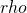is the air density at altitude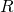.  The fluid atmosphere refracts sunlight according to Snell’s law.  The  column depth or path length of sunlight then becomes:

(3)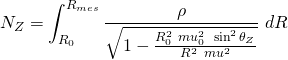The equation is the column depth of the atmosphere with a correction factor in the denominator for the the refraction of light, where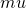is the air’s index of refraction at altitude R andis the apparent zenith angle.  Again, to get the air mass,must be normalized by the column depth when.

#### The Standard Atmosphere

The atmospheric air mass equation relies on air density as a function of altitude.  This is estimated using a temperature profile combined with the ideal gas law (which expresses density in terms of pressure and temperature), along with the assumption of hydrostatic stability (which defines density in terms of pressure).

The problem of the temperature profile can only be determined by solving equations for the fluid dynamics of the atmosphere, coupled with knowledge of the radiative energy in the atmosphere and given appropriate boundary conditions.  This is the fundamental challenge of numerical weather prediction for which there are standard models (WRF, MM5, etc.) and gridded data sets for both historic or forecast time frames.

#### Applying the Standard Atmosphere

For the temperature profile, the atmosphere is assumed to have a discrete number layers within which the temperature changes  linearly with altitude.  In any given layer n, the temperature is

(4)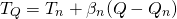whereis the slope of the temperature rise with altitude within the layer n,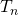is the temperature at the bottom of that layer,is the height (defined below), and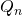is the height of  the bottom of the layer.  This is the methodology used in the U.S. Standard Atmosphere, 1972, wherein a “standard atmosphere” appropriate for the continental United States is defined.  A calculator for the standard atmosphere coded in R appears at the end of this post.

In practice, it is necessary to adjust the standard atmosphere to obtain specific temperatures and air mass profiles for the location of interest.  The bottom eight temperature layers correspond roughly to the troposphere, stratosphere, and mesosphere.  The top or eighth layer is at 100 km above mean sea level.  In order to generalize the work to make it more appropriate for any latitude and any season,  then the values defining the lowest two layers (the troposphere and lower stratosphere) are most important.

Specifically, let the boundary between those two layers (the tropopause;vary as a function of time of year and latitude.  The same thing goes for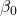, the temperature fall-off in the first layer.  The bottom of the first layer (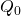) is set to the heightof the observer’s location.  The boundary condition temperature at the bottom of first layer (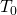) is set to the actual local temperature at the observer.  The condition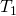equals the temperature at the top of the first layer, i.e., as determined from the  above expression for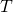with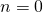and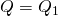.  Finally,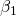is set by forcing the temperature at the top of the second layer to match(fixed at some value as reported by the Qatar Meteorology Department, as opposed to 216.65 K, the value in the U.S. Standard Atmosphere).